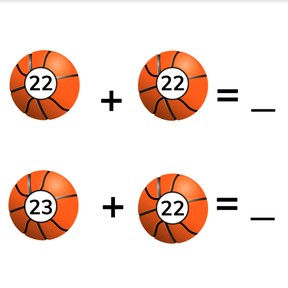Addition to 100 with one more or one less

# Addition to 100 with one more or one less8,000 schools use Gynzy92,000 teachers use Gynzy1,600,000 students use Gynzy

## General

Students learn to add to 100 by adding with one more or one less to reach their total.

2.NBT.B.5

## Relevance

Discuss with students that it is useful to be able to add with one more or one less. If you know when you add one more or take one away, you don't need to redo calculations on sets of addition problems.

## Introduction

Play bingo with the students. Students write nine numbers on their cards with numbers from 70-100. You say a number and students are allowed to cross off that number, or its neighbor numbers on their bingo card. Say numbers until a students says "Bingo". Then show the flags on the board and ask which numbers are missing. Ask students to explain how they know which numbers are missing. Erase the grey boxes to show the correct answers.

## Development

Check that students are able to add to 100 with one more or one less by asking the following questions/giving the following exercises:
- What must you look at to know if you need to add one more or take one away?
- Calculate the following: 48+2= and 48+3 =
- Calculate the following: 10+63 =, 9+63 =, 8+63 =

## Guided practice

Students first practice adding with one more or one less with sets of two addition problems. Students are then asked to solve sets of three addition problems.

## Closing

Discuss with students that it is important to be able to add to 100 with one more or one less so you are able to easily solve sets of addition problems without calculating each one separately. Show the set of addition problems on the interactive whiteboard and ask students to calculate the answers. Ask how they solved the problems. Erase the grey boxes to reveal the answers.

## Teaching tips

Students who have difficulty with adding with one more or one less can be supported by using MAB blocks or manipulatives. Ask them to set out the addition problem of the first set and then to solve a problem with one more by adding one more block. Repeat this with different addition problems and then switch to demonstrating addition problems with one less.

## Instruction materials

Optional: MAB-blocks or manipulatives

### The online teaching platform for interactive whiteboards and displays in schools

• Save time building lessons

• Manage the classroom more efficiently

• Increase student engagement